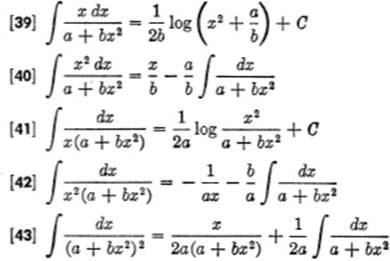﻿ ﻿Tables - The Calculus Primer

## The Calculus Primer (2011)

### Tables

INTEGRALS

A. Standard Elementary Formsdx = x + Ca dv = adv(du + dvdw) =du +dvdw[7a]ev dv = ev + Csin v dv = − cos v + Ccos v dv = sin v + Csec2 v dv = tan v + Ccsc2 v dv = − cot v + Csec v tan v dv = sec v + Ccsc v cot v dv = − csc v + Ctan v dv = log sec v + C = − log cos v + Ccot v dv = log sin v + Csec v dv = log (sec v + tan v) + Ccsc v dv = log (csc v − cot v) + CB.Forms Containing (a + bx)C.Forms Containing (a2 + x2), (a2x2), (a + bx2)D.Forms ContainingE.Forms ContainingF.Forms ContainingG.Forms ContainingH.Forms Containing Trigonometric FunctionsI.Forms Containing Exponential and Logarithmic FunctionsVALUES OF ex AND e−x

VALUES OF ex FROM x = 0 TO x = 5.9VALUES OF e−x FROM x = 0 TO x = 5.9COMMON LOGARITHMS﻿